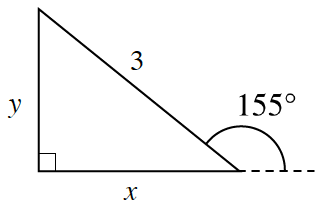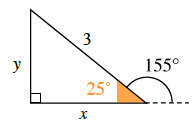Home > A2C > Chapter 1 > Lesson 1.2.1 > Problem1-68

1-68.A $3$-foot indoor children’s slide must meet the ground very gradually and make an angle of $155°$, as shown in the diagram at right. Find the height of the slide ($y$) and the length of the floor it will cover ($x$).

Review the trigonometric ratios from the Math Notes box in this lesson. Which angle represents "$θ$" for those equations?

In order to produce the correct value, the inside angle must be used. See the diagram below.